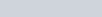ChemTalk

# Alpha, Beta, and Gamma Decay## Core Concepts

In this article, we explore the phenomenon of radioactive decay and its most common types: alpha, beta, and gamma decay.

## Topics Covered in Other Articles

“Radioactive decay” refers to a group of reactions involving the nucleus of an atom changing in some form. Radioactive decay occupies an important place in nuclear chemistry, or the branch of chemistry concerned with the change of nuclei, along with nuclear fusion and nuclear fission. There are three main radioactive decay reactions: alpha decay, beta decay, and gamma decay.

As implied by the term “decay”, radioactive decay often involves the gradual disappearance of a substance. Indeed, in alpha and beta decay, radioactive nuclei (or “radionuclides”) change their number of protons, altering their atomic number, and becoming a different element. In gamma decay, the nuclei only change from their excited to ground state.

All forms of radioactive decay involve the release of some particle from the nuclei. Chemists call this release “radiation”. When in contact with non-radioactive atoms, this radiation can extract or displace an electron, leaving the substance “ionized”. The ionizing potential of these particles makes them dangerous to biological systems. To learn about the chemistry of radioactive particles and their benefits and risks to humans, check out this article.

In both alpha and beta decay, this particle has mass, while in gamma decay, the particle is a massless photon.

Alpha decay involves the emission of an alpha particle from the parent nuclei. This alpha particle is essentially the nucleus of helium or a He2+ ion. Thus, alpha particles have two protons and two neutrons. With the release of the alpha particle, the parent nucleus thus loses 4 atomic mass units. Further, from the loss of protons, the atomic number decreases by two.

One isotope capable of alpha decay is radium-226, which appropriately decays into radon-222.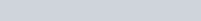Radium-226 has a half-life of 1602 years. This means that after 1602 years, half of a given amount of radium-226 will have alpha decayed into radium-222.

Beta decay (β-) involves the emission of a beta particle from the parent nuclei. This beta particle is a high-energy electron. However, rather than originating from the atom’s electron cloud, this electron comes from one of the nuclei’s neutrons. Intriguingly, with the loss of this electron, the neutron converts into a proton. This increases the atomic number by one while the mass remains constant.

One isotope capable of beta decay is bismuth-234, which decays into polonium-234.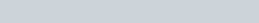Bismuth-234 has a halflife of 19.9 minutes.

#### The Electron Antineutrino

In addition to electrons, beta decay also gives off anti-matter particles that chemists call electron antineutrinos (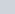).

Both electrons and electron antineutrinos count as “leptons”, a variety of subatomic particles. According to quantum theory, the total number of leptons in the universe must remain constant or “balanced” with their antimatter counterparts. Thus, since beta decay creates an electron that didn’t previously exist, it must also create an electron antineutrino to cancel out.

Thus, including the electron antineutrino, the beta decay of bismuth-234 looks like this: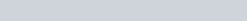We can also generalize beta decay as one neutron converting to one proton, an electron, and an electron antineutrino: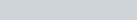#### Positron Emission

The version of beta decay we’ve thus far discussed is more specifically called “beta-minus decay”, referring to the negative charge of the emitted electron. There exists also a much rarer version called “beta-plus decay” which you can think of as the opposite process.

Beta-plus decay releases a positron as a beta particle. Essentially, the positron behaves as a positively charged electron and, like the electron antineutrino, also counts as antimatter. To follow the conservation of leptons, beta-plus decay also releases an electron neutrino (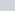), which is matter. Further, beta-plus decay results in a proton converting to a neutron, resulting in a decrease of one atomic number.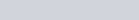One isotope capable of beta-plus decay is carbon-11, which decays into boron-11: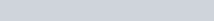As mentioned before, gamma decay doesn’t involve the conversion of one element to another. Instead, in gamma decay, when a nucleus reaches an energized state, it gives off light photons. Nuclear chemists typically call these photons “gamma rays”. Occasionally, you may also see alpha and beta particles described as “alpha rays” and “beta rays”, but remember, only gamma rays involve light.

Often, gamma decay occurs as the result of alpha or beta decay. When a nucleus gives off an alpha or beta particle, a gamma ray is also emitted. Alpha or beta decay also excites the resulting nucleus, which then emits another gamma ray to return to the ground state.

For instance, when uranium-238 performs alpha decay, it releases a gamma ray photon.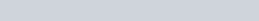This newly-formed thorium atom is left in the excited state immediately following the alpha decay. To return to the ground state, it must then give off a second gamma ray.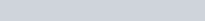The same pattern of gamma ray emission occurs with beta decay. Take this example with the beta-minus decay of cobalt-60: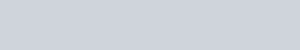Gamma decay tends not to have a half-life, since gamma ray emission basically happens instantly.

While alpha, beta, and gamma decay compose the vast majority of radioactive decay reactions, some rarer nuclear decay reactions do occur. For instance, a nucleus can emit a single proton or neutron. This tends to occur in the excited state immediately following alpha or beta decay. Typically, a nucleus only emits a proton or neutron when the proportion of protons to neutrons is extremely imbalanced.

Other instances involve an extremely high change in stability from the subsequent loss of a proton or neutron. For instance, oxygen-17, produced from the beta decay of nitrogen-17, tends to instantly undergo neutron emission to reach the super-stable oxygen-16.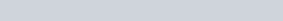Radioactive nuclei can also undergo what nuclear scientists call “cluster decay”, which involves the emission of a nuclear chunk larger than an alpha particle. Typically, cluster decay occurs in a small proportion of radioactive nuclei that generally participate in alpha decay. One radionuclide capable of cluster decay is radium-224, which emits carbon-14 to then transform into lead-210.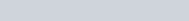Problem 1

Astatine-219 (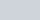) undergoes beta decay followed by two alpha decays. What isotope is produced?

Problem 2

You have a sample of 15 astatine-219 isotopes. Each undergoes the same decay chain as described in (1). How much of each radioactive particle is produced during this radioactive decay?

## Radioactive Decay Practice Problem Solutions

1: Lead-211 (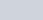)

2: